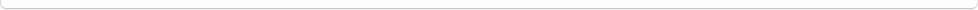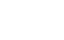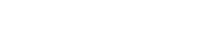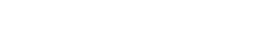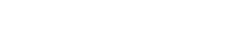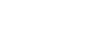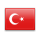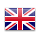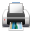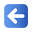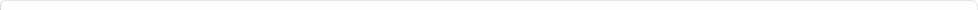Description of Individual Course Units
 Course Unit Code Course Unit Title Type of Course Unit Year of Study Semester Number of ECTS Credits 180104004100 ENGINEERING MATHEMATICS Compulsory 2 4 5
Level of Course Unit
First Cycle
Objectives of the Course
The aim of the course is to introduce students to the application areas of differential equations in engineering and their importance with examples. To present modern, concrete and field-specific examples and problem sets presented from nature and life in addition to theory in a language that the student can easily understand.
Name of Lecturer(s)
Dr. Muhammet Raci AYDIN
Learning Outcomes
 1 Will be able to formulate mathematical models of various problems. 2 Will be able to solve the model by using some analytical, qualitative and partial numerical methods. 3 They can determine the solution of a well-defined problem studied in the course.
Mode of Delivery
Daytime Class
Prerequisites and co-requisities
no prerequisite
Recommended Optional Programme Components
no prerequisite
Course Contents
First order differential equations, higher order differential equations, vectors and 3-space, vector valued functions, partial derivatives, multiple integrals and integral calculus in vectors
Weekly Detailed Course Contents
 Week Theoretical Practice Laboratory 1 General definitions; first order differential equations. separable equations. linear equations, mathematical models. 2 First order differential equations. Solution curves without solution, Euler's method. 3 Vectors in 2D space and Vectors in 3D space. dot product. vector multiplication. 4 Lines in 3D space. planes, cylinders and spheres. fourth-order surfaces 5 Vector-valued functions, calculus of vector functions, motion on a curve, curvature and acceleration. 6 Partial derivatives, multivariable functions, limit continuity, partial derivatives, linearization and differentials, chain rule. 7 chain rule, directional derivatives, tangent plane and normal line, extremums of multivariable functions, least squares method, lagrange multipliers. 8 Multiple integrals, double integrals, sequential integrals, calculation of double integrals, center of mass and moments, double integrals in polar coordinates. 9 Surface area, triple integrals. 10 triple int., variable replacement in multiple integrals in other coordinate systems. 11 Line integrals, curve integrals of vector fields, path independence, Green's theorem. 12 parametric surfaces and area, surface integrals, curl and divergence, stokes theorem, divergence theorem. 13 first order full diff. equations, homogeneous diff. equations, inhomogeneous linear equations. 14 mathematical models, power series solutions 15 midterm
Calculus II Early Transcendentals. Dennis G. ZILL, Warren S. WRIGHT, Jones. 4th edition.
Planned Learning Activities and Teaching Methods
Assessment Methods and Criteria
 Term (or Year) Learning Activities Quantity Weight Midterm Examination 1 100 SUM 100 End Of Term (or Year) Learning Activities Quantity Weight Final Examination 1 100 SUM 100 Term (or Year) Learning Activities 40 End Of Term (or Year) Learning Activities 60 SUM 100
Language of Instruction
Turkish
Work Placement(s)
no prerequisite